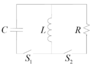# Voltage across an inductor after flipping a switch

• Eitan Levy

#### Eitan Levy

Homework Statement
In the circuit below the initial charge of the capacitor is Q. First, we shut S1 (S2 remains open) and wait for the first time in which the energy of the capacitor is minimal.
Then, we shut S2 and open S1 simultaneously. What is the voltage on the inductor right after the change?
Relevant Equations
ω=1/sqrt(LC)
I(t)=-ωQsin(ωt)
When the energy of the capacitor is minimal it has no charge therefore the voltage on it is 0. That means that the voltage on the inductor is 0 as well at the moment.
The part that confuses me is: why isn't the correct answer 0? How can a voltage change in a single moment?
Furthermore I am confused because in the solution, they used the fact that the current in the inductor before the change was Q/sqrt(LC) and therefore got the answer QR/sqrt(LC) . Why can the voltage shift like that but the current can not? How can we know what will remain constant (for a moment, in this case the current), and what may change instantly?

#### Attachments

•Capture.PNG
6.5 KB · Views: 76

It's ok for voltage and current to change suddenly in a circuit. A simple example is a circuit with a battery, switch and resistor. When the switch is closed, there is suddenly a voltage across the resistor and a current through the resistor. (Every circuit is going to have some inductance which prevents the current from changing truly instantaneously. But, if the inductance is very small, the current can change essentially instantaneously.)

At the moment the switching occurs in your circuit, is there any energy in the circuit? Can the energy change suddenly?

It's ok for voltage and current to change suddenly in a circuit. A simple example is a circuit with a battery, switch and resistor. When the switch is closed, there is suddenly a voltage across the resistor and a current through the resistor. (Every circuit is going to have some inductance which prevents the current from changing truly instantaneously. But, if the inductance is very small, the current can change essentially instantaneously.)

At the moment the switching occurs in your circuit, is there any energy in the circuit? Can the energy change suddenly?
I figured it will have something to do with energy. As far as I know energy cannot change instantly. The energy is 0.5LI^2, therefore I cannot change suddenly?

I figured it will have something to do with energy. As far as I know energy cannot change instantly. The energy is 0.5LI^2, therefore I cannot change suddenly?
Yes. Just before the switching, the inductor has the energy ##\frac 1 2 L I^2##. This energy must still be in the LR circuit after the switching (unless the energy was dissipated in sparking in the switches, which we assume doesn't happen.)

Yes. Just before the switching, the inductor has the energy ##\frac 1 2 L I^2##. This energy must still be in the LR circuit after the switching (unless the energy was dissipated in sparking in the switches, which we assume doesn't happen.)

One question about this - does the energy must be continuous in the entire circuit or in the inductor itself? Can the energy completely "disappear" from the inductor instantly, while moving somewhere else?

Can the energy completely "disappear" from the inductor instantly, while moving somewhere else?
I don’t believe that this is possible.

•Eitan Levy